Math Concepts

# Sequences and Series

596 views

 1 Introduction 2 Sequences 3 Sequences in real life 4 Series Definition 5 Sequence vs Series 6 Progression Definition 7 Types of Sequences 8 Common Sequences and Examples. 9 Summary 10 FAQs

16th September 2020

## Introduction

Sequences and series are a very relevant part of our lives.

Their applications can be seen in numerous fields and they help understand various patterns around us.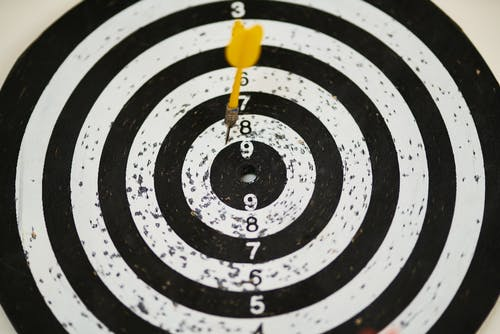But before we delve into specific progressions like arithmetic and geometric, let us familiarize ourselves with the basic terms and concepts.

### Define consecutive

Consecutive objects mean that they follow one after the other in an ordered succession or sequence. For eg, in natural numbers, $$5$$ and $$6$$ are consecutive numbers.

### Successive Definition

It is the synonym of consecutive. It also indicates the following elements one after the other or many others.

For example, $$3, 4, 5$$ …. are elements successive to $$2$$

## Sequences and Series - PDF

If you ever want to read it again as many times as you want, here is a downloadable PDF to explore more.

Sequences are lists of objects or numbers that are observed to have an order or follow a particular pattern or function.

Sequences have been known to be both finite and infinite.

Each number of such sequences is called an element or a term.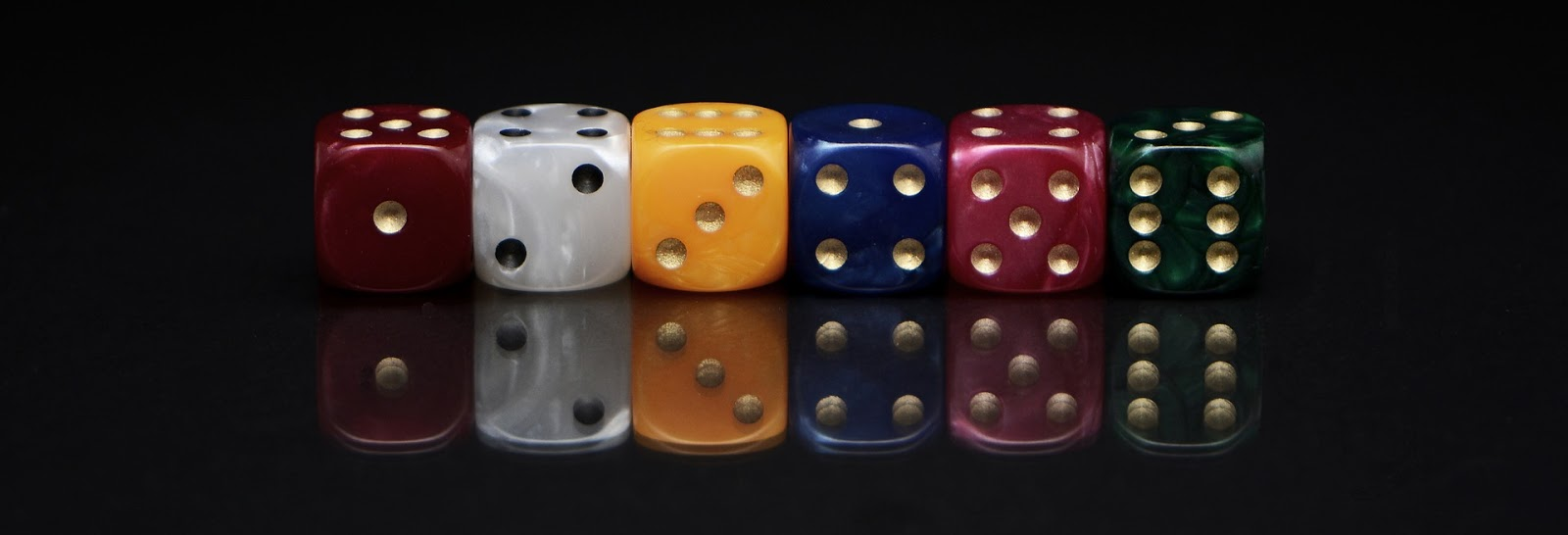The collection of ordered objects which go on forever are called infinite sequences. Else they are finite sequences.
Examples:

$$[ 2, 4, 6, 8, 10 …………]$$---> even numbers (infinite sequence)
$$[3... 9…27... 81…….]$$---> powers of $$3$$ (infinite sequence)
$$2, 3, 5, 7$$ ---> prime numbers below $$10$$ (finite sequence)

Many other logical sequences that will be discussed more a little further on.

### Limit of a sequence

The limit of a sequence is the value the entire sequence tends to finally. This could be both infinite or finite value. If the limit is a finite value and actually observed to exist, the sequence is defined as convergent since it   converges to a definite value, otherwise, it is divergent.

## Can you see sequences in real life?

The world around us is full of patterns and orders.

We see various logical sequences in nature from time to time.

In fact, everything can be brought down to some order and reason.

When we speak about how humans continue to habitat the earth and our existence, we refer back to DNA sequences.

This is because the natural processes follow certain rules which provide us with an immeasurable number of interesting sequences hidden.Looking at smaller scale observations, let us take an example of a game we all are familiar with- Jenga. To play Jenga, we stack the blocks in a particular order.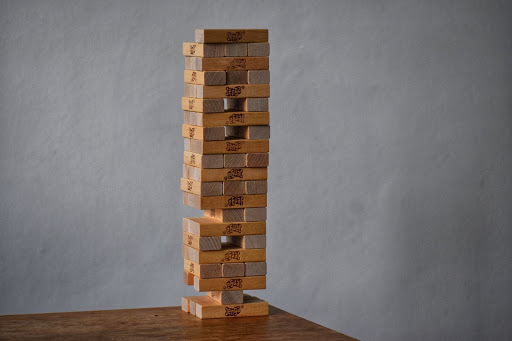Once we begin playing the game, we start disrupting the order and it becomes just another collection of blocks randomly stacked up.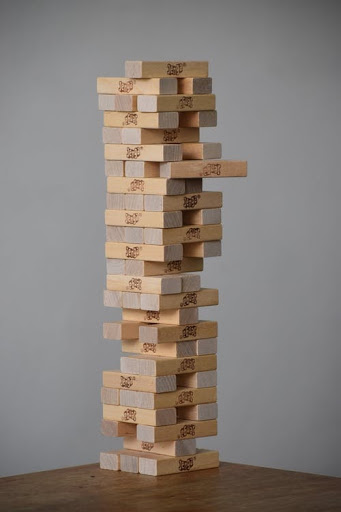Hence the sequence was broken.

We can observe sequences even in nature, astronomy, and huge natural phenomenons like the Lunar sequence, the sequence of planets in the Milky Way. The sequence of the water cycle, nitrogen cycle, life cycle of a butterfly, etc. It has been proven that nature prefers a natural state of order.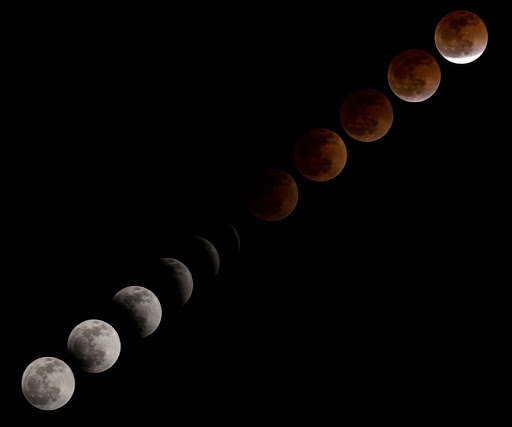Everything from how many petals are present in a flower to how the planets revolve around the sun involves sequences.

Earthquakes, water flow, population, financial markets, you name it.

Mathematically, concepts of multiples, powers, BODMAS sequence, etc all have ordered sequences.

## Series Definition

A series is simply the sum of a given sequence.

Note: The sum of a particular part of a sequence is called its partial sum. It is not to be confused with a series.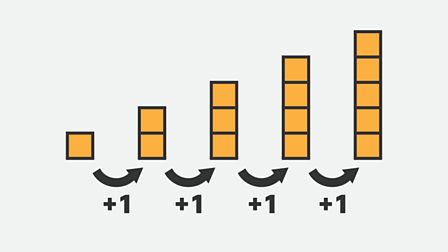Example 1

\begin{align}+ &2 + 3 + 4 + .......\\&3 + 6 + 3 + 6 + 3......\\&1 + \frac{1}{2} + \frac{1}{4} + \frac{1}{8}....\end{align}

## Sequence vs Series

One of the most common doubts that pop in our heads is Series vs Sequence. So,

### What is the difference between a sequence and a series?

As mentioned above,

A sequence is just a list of ordered objects following a pattern or some rules generally. In this, the order matters a lot.
$$1 \,4\, \,8\, 12\, 16\, ….$$Is one sequence while,
$$16\, 12\, 7\, 4\, 1\,…….$$ is a separate sequence.

A sequence can be both finite and infinite.

They are not the same since the rule for the successive or consecutive elements are different.

On the other hand, a series is a sum of a partial part of an infinite sequence and generally comes out to be a finite value itself.

Hence, \begin{align}1 + 4 + 8 + 12 + 16 = 41\end{align} is one series and
\begin{align}16 + 12 + 8 + 4 + 1 = 41\end{align} yields the same sum

Which implies, the rearrangement of these elements will not change the resulting sum hence, the order doesn't hold that much importance.

So once we analyze sequence vs series we can see the clear contrast from the above discussion.

## Progression Definition

Progression refers to a sequence in which each element is related to its predecessor by a uniform rule throughout. It is synonymous with sequencing.

So, instead of arithmetic sequence, geometric sequence, harmonic sequence these are also called arithmetic progression, geometric progression, and harmonic progression alternatively.## Types of Sequences

There are various kinds of series and sequences on the nature of terms, the limit of the sequence, the rule followed, etc. But we can talk about some special sequences which are most commonly seen and talked about:

1. Arithmetic Sequence
2. Geometric Sequence
3. Harmonic Sequence
4. Fibonacci Sequence

There can be many other sequences but these hold some importance in our day to day lives and practical applications.

Arithmetic Sequence is a sorted list of numbers where every pair of successive terms have a uniform and constant common difference. To learn more about this, visit Arithmetic Sequence.

Geometric Sequence on the other hand is a sorted list of numbers where every pair of successive terms have a uniform and constant common ratio. This means the division of two consecutive terms will give the same value. To learn more about this, visit Geometric Sequence.

Harmonic Sequence is a special sequence where the reciprocals of the terms seem to be in an arithmetic arrangement and have a common difference. To learn more about this, visit Harmonic Sequence.

Fibonacci Sequence is a very interesting ordered arrangement of numbers where the sum of two successive terms gives the value of the next in order.
\begin{align}1\, 1\, 2\, 3\, 5\, 8\, 13\,………\end{align}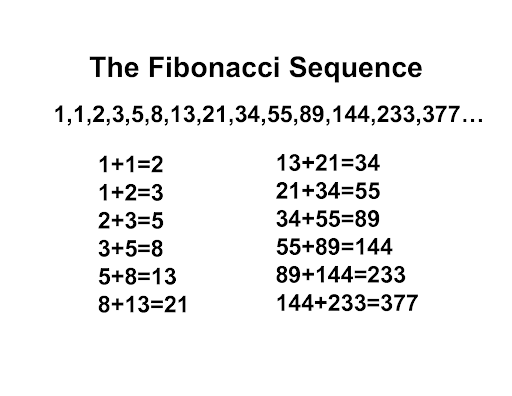For a look at other common sequences, let us move onto the next section.

## Common Sequences and Examples

 Example 1

What is the next number?

\begin{align}1\, 1\, 2\, 4\, 3\, 9\, 4\,\end{align}

Solution:

At first glance, this will seem like a random arrangement. But notice how $$1$$ squared gives $$1, 2$$ squared gives $$4, 3$$ squared gives $$9$$. So the square of a number comes next in line, with the next natural number following it.

\begin{align}1, 1\times1, 2, 2\times2, 3, 3\times3….\end{align}
Using that logic, the next term after $$4$$ should be $$4$$ squared.

 Hence, the answer is square of $$4 = 16$$.
 Example 2

\begin{align}1\, 3\, 4\, 7\, 11\, 18\, 29\, ?\end{align}

Solution:

This particular series is quite similar to a kind of series we have spoken about in the last section. Notice how the sum of $$1$$ and $$3$$ gives $$4$$ and $$4$$ and $$7$$ gives $$11$$.
So,

\begin{align}&1 + 3 = 4\\&3 + 4 = 7\\&4 + 7 = 11\\&7 + 11 = 18\\&11 + 18 = 29\end{align}
And so,

 Next will be \begin{align}18+ 29 = 47. \end{align}
 Example 3

Which of the following is an arithmetic sequence?

\begin{align}&\rm{(a)}\,2\,4\,8\,16\,32\,\\&\rm{(b)}\,3\,7\,12\,18\,25\,\\&\rm{(c)}\,4\,8\,12\,16\,20\,\\& \rm{(d)}\,1\,3\,5\,8\,13\,20\end{align}

Solution:

This sequence is the ordered arrangement  of the successive powers of $$2$$
\begin{align}2, 2\times2, 2\times2\times2, 2\times2\times2\times2……\end{align}
Hence, this is not an arithmetic sequence. Rather, it is a geometric one.

Once we resolve the difference between two terms, we find out the difference between the terms are also incrementing at a constant rate.
\begin{align}7 - 3 &= 4\\12 - 7 &= 5\\18 - 12 &= 6\end{align}and so on.

 This one is an AP whose difference between two successive terms is $$4$$. This is a random set of numbers.

## Summary

This blog explored the different basics behind the more advanced progressions and how the language of the terms used also makes a difference in problems of series and sequence.

A comparison of Sequence vs Series yields  the result that sequences are only a list of ordered elements while a series is the actual summation.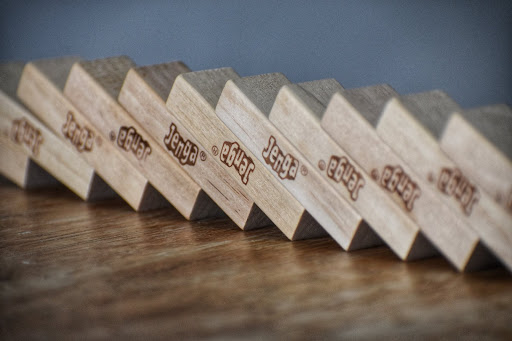Then there are also terms like successive, consecutive, progression and limit of a sequence that are used recurring, and hence an understanding will help going forward.

Cuemath, a student-friendly mathematics platform, conducts regular Online Live Classes for academics and skill-development and their Mental Math App, on both iOS and Android, is a one-stop solution for kids to develop multiple skills. Know more about the Cuemath fee here, Cuemath Fee

## Define consecutive?

Consecutive objects mean that they follow one after the other in an ordered succession or sequence. For eg, in natural numbers, $$5$$ and $$6$$ are consecutive numbers.

## What is the difference between a sequence and a series?

A sequence is just a list of ordered objects following a pattern or some rules generally. In this the order matters a lot.

On the other hand, a series is a sum of a partial part of an infinite sequence and generally comes out to be a finite value itself.
The order doesn't hold that much importance.

## Types of sequences?

• Arithmetic Sequence
• Geometric Sequence
• Harmonic Sequence
• Fibonacci Sequence

## External References

Related Articles
GIVE YOUR CHILD THE CUEMATH EDGE
Access Personalised Math learning through interactive worksheets, gamified concepts and grade-wise courses